# Scripting in CIAO

## Introduction

This talk/demonstration aims to introduce you to using the Python programming language, to enhance your productivity when analyzing data using CIAO. It is not an introduction or tutorial to Python; for that I suggest either talking to the grad students in your department, the pythonusers email list here at the CfA, or

(I found 'Dive Into Python' to be helpful but unfortunately the web site no longer exists).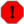Beware snakes bearing apples; in particular apples marked with a 3! CIAO 4.2 comes with Python version 2.6, which is very common, but is slightly incompatible with the latest version of Python (version 3). Note that most documentation will be about version 2.x of Python, since many of the important modules have not been upgraded yet, so you should be fine.

It's also useful to know about:

NumPy (Numerical Python)
which provides much of the capabilites that make Python a useful language for scientific data analysis.
AstroPython
a new site for Astronomers who use Python.

## What is scripting?

Here's one definition - the ability to make the computer do all the boring, repetitive, computational tasks, such as (but not limited to)

• easily generate a plot so that when you show it to your boss and they ask "Can you just twiddle this label a bit and add on Joan's data?" you don't have to redo everything;
• regenerate all the results and plots for you paper because the referee asked you what difference the latest CALDB makes to your analysis;
• repeat an analysis task you developed for one dataset with others you find in the archive;
• and develop routines that perform common (and not-so-common) tasks that you can use from Sherpa and ChIPS (i.e. interactively).

The aim is to spend less time writing these scripts and routines than it would to do it manually!

## A very simple demonstration: plotting data

We shall begin with creating a simple plot in ChIPS, using the interactive application, and then work through various steps and approaches for "scripting" this task.

Here's the data file we want to plot; it contains K-band magnitudes of a bunch of brightest-cluster galaxies:

```unix% cat bcg.txt
# name z Lx pos mk alpha
ms0002_8              0.116   1.643   1   12.38   7.830333e-01
ms0007_2              0.050   0.517   1   11.11   4.452510e-01
...
```The CIAO tools can read FITS or ASCII files using the Data Model syntax; for instance to get the statistics of the lx column you can use dmstat:
```unix% dmstat "bcg.txt[cols lx]" sig-
Lx
min:	0.04 	      @:	21
max:	19.976 	      @:	18
mean:	3.5656896552
sum:	310.215
good:	87
null:	0
```
We will come back to calculating these numbers ourselves.

Starting ChIPS, we can quickly create a basic plot of the data:

```unix% chips
Welcome to ChIPS: CXC's Plotting Package
-----------------------------------------
CIAO 4.2 Monday, November 30, 2009

chips-1> make_figure("bcg.txt[cols z,lx]")
chips-2> set_curve(["line.style", "noline", "symbol.style", "circle"])
chips-3> from chips_contrib import *
chips-4> ylog()
chips-5> set_plot_ylabel("L_x [10^{44} erg s^{-1}]")
chips-6> add_label(0.6, 0.1, "A spiffy plot", ["size", 18])
chips-7> set_label(["halign", 0.5])
chips-8> ,ahelp print_window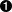chips-9> print_window("bcg-z-lx.png")
```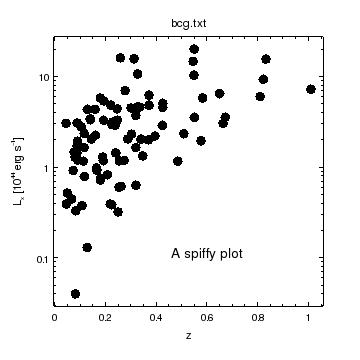The "," before a command makes ChIPS or Sherpa (really it is ipython) add quotes around the first argument (here print_window). We are also taking advantage of the "auto-add-a-bracket" magic to avoid having to type
`ahelp("print_window")`
I haven't taken advantage of this "auto-add-a-bracket" magic elsewhere because it is not supported when you write "scripts", as we do below.

These are all Python commands, provided by these ChIPS module (apart from ylog and the ,ahelp lines). The chips application provides a Python "interpreter" (a so-called REPL if you want to get fancy) at which you can enter commands. In this example we have not tried anything too fancy.

There are a range of ways we can automate the creation of this plot, which we explore below.

### Using the ChIPS and Sherpa state mechanisms

This is not really scripting, but it may provide all you need for this simple situation. There are several ChIPS and Sherpa commands that will save the current session, allowing you to re-create it at a later time or to send it to a collaborator.

script (ChIPS and Sherpa)

This command will save all the commands you have entered at the ChIPS or Sherpa prompt to a text file.

After

`chips> script("example1.py")`

the file can be loaded into a ChIPS session by saying one of:

`unix% chips example1.py`

to start a new session, or

`chips> execfile("example1.py")`

to load it into an existing session. The same commands work in Sherpa.

save_state (ChIPS) and save (Sherpa)

These create binary-format files (so it is not human readable) which allow you to recreate the ChIPS visualization or Sherpa session in another session, even on a different machine. The files can be used on any operating system on which CIAO runs. The binary files include all the data needed to recreate the plots (ChIPS) or the fits (Sherpa), so you do not also need to package the original data files.

After

`chips> save_state("example1.state")`

the file can be loaded into a ChIPS session by saying:

`chips> load_state("example1.state")`

The equivalent commands in Sherpa are:

`sherpa> save("example1.save")`

and

`sherpa> restore("example1.save")`The file name need not end in .state or .save.
make_script (ChIPS) and save_all (Sherpa)

These create a file of Python commands which, when run, will re-create the ChIPS visualization or Sherpa fits.

If necessary, the make_script command will save out the data files to FITS files, but it can refer to existing files (e.g. if you used the high-level commands like make_figure to create the plots). Although the output is a set of Python commands, it is unlikely to look like the commands you entered to create the visualization in the first place!. The save_all command does not save data (so it will not work fully with data that has been created or manipulated rather than read in straight from a file).

After

`chips> make_script("example1.py")`

the file can be loaded into a ChIPS session by saying one of:

`unix% chips example1.py`

to start a new session, or

`chips> execfile("example1.py")`

to load it into an existing session.

The equivalent commands in Sherpa are:

`sherpa> save_all("example1.py")`

and

`sherpa> execfile("example1.py")`

### Simple copy and pasting

The commands you used can be copied into a text file and then loaded into ChIPS or Sherpa. For instance:

1. `from chips_contrib import *`
2. `# Load in the data`
3. `make_figure("bcg.txt[cols z,lx"])`
4. `# Adjust the attributes/look of the plot`
5. `set_curve(["line.style", "noline", "symbol.style", "circle"])`
6. `ylog()`
7. `set_plot_ylabel("L_x [10^{44} erg s^{-1}]")`
8. `add_label(0.6, 0.1, "A spiffy plot", ["size", 18])`
9. `set_label(["halign", 0.5])`
10. `# Print out`
11. `print_window("bcg-z-lx.png", ["clobber", True])`
12. `print "Created: bcg-z-lx.png"`

We have added comment lines (those that begin with '#'), moved the import of the chips_contrib module to the start of the file, set the clobber attribute to True in the print_window call, and told the user what we have done with the print line.Unlike the interactive session, all functions must include brackets around their argument lists (even if this is empty), and string values must be quoted. The print command is "special" (and is one of the things that will change in Python 3).Whitespace is important in Python, so don't start a line with spaces, or tabs, unless you know what you're doing.

This file can then be loaded using one of the following approaches:

1. From within ChIPS or Sherpa:

`chips> execfile("spiffy-plot.py")`
2. When starting ChIPS or Sherpa:

```unix% chips spiffy-plot.py
...
Created: bcg-z-lx.png
chips-2>
```

This will leave you at the prompt, which is useful if you want to use this as a starting point for further work.

3. Use the "batch" mode when starting ChIPS or Sherpa:

```unix% chips -b spiffy-plot.py
...
Created: bcg-z-lx.png
unix%
```

Once all the commands have been processed, ChIPS (or Sherpa) will exit, leaving you back at the prompt. This also turns off the ChIPS "set_window"redraw" mode, so you will not see an on-screen version of the ChIPS window flash up and then disappear.

### Making a more "script-like" script

The previous section showed our first script, and used ChIPS to execute it. Here we will change the script so that it can be run just like a tool, and include simple processing of command-line arguments.

We start the file with a "shebang line" which tells the system what program we want to run/interepret the code (in this case python), so that the top of the file now looks like:

1. `#!/usr/bin/env python`
2. `# Load in the modules that the ChIPS application provides for us`
3. `from pychips import *`
4. `from pychips.hlui import *`The set of modules to load depends on the application; in this case use ahelp chips or ahelp sherpa to find out what needs to be loaded.

We have also had to explicitly load the ChIPS modules (the ChIPS application loads these in for us, which is why you haven't seen them before). The rest of the file matches spiffy-plot.py. If given the excutable bit - e.g. via chmod u+x spiffy-plot-script - then we can just say

`unix% ./spiffy-plot-script`

to run it, otherwise we have to use

`unix% python spiffy-plot-script`

We now decide that we want to give an arbitrary file name for the input and output: first we try using simple Python commands, then will show an example of using the CIAO parameter interface, using the paramio module.

#### Simple python argument parsing

1. `#!/usr/bin/env python`
2. `import sys`
3. `from pychips import *`
4. `from pychips.hlui import *`
5. `import chips_contrib as ctrb`
6. `def usage(progname):`
7. ` "Error out with a usage message"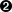`
8. ` print "Usage: %s <infile> <outfile>" % progname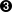`
9. ` sys.exit(1)`
10. `def plotfile(infile, outfile):`
11. ` """Plot up the lx versus z columns of infile`
12. ` and create a PNG version in outfile."""`
13. ` # Load in the data`
14. ` make_figure("%s[cols z,lx]" % infile)`
15. ` # Adjust the attributes/look of the plot`
16. ` ci = ChipsCurve()`
17. ` ci.line.style = "noline"`
18. ` ci.symbol.style = "circle"`
19. ` set_curve(ci)`
20. ` ctrb.ylog()`
21. ` set_plot_ylabel("L_x [10^{44} erg s^{-1}]")`
22. ` add_label(0.6, 0.1, "A spiffy plot", ["size", 18, "halign", 0.5])`
23. ` ofile = "%s.png" % outfile`
24. ` print_window(ofile, ["clobber", True])`
25. ` print "Created: %s" % ofile`
26. `# If run as a script, call the routine`
27. `if __name__ == "__main__":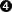`
28. ` # Check script was called correctly`
29. ` if len(sys.argv) != 3:`
30. ` usage(sys.argv)`
31. ` # Call the routine whilst`
32. ` # emulating the "batch-mode" of ChIPS`
33. ` set_preference("window.display", "false")`
34. ` plotfile(sys.argv, sys.argv)`The chips_contrib module has been loaded into a specific "namespace" - in this case ctrb - rather than the default one. Routines from this module can only be accessed via this label, so we now have to say ctrb.ylog(). For this example there is no benefit in doing this, but it can be helpful when you have many modules loaded, and want to know what module is being used, or to avoid name clashes.Documentation of a routine is specified as an optional string following the routine name. A triple quote - """ - indicates a multi-line string.Here we use C-like format specifiers (e.g. %s) to include variables into strings (also known as string formatting or interpolation). This is another area that is different in Python 3.This line is intended to make sure that the code only gets evaluated when the file is run as a "script", rather than loaded as a module. Don't worry about it.

Here we see our first example of white space/indentation where blocks of related code - in this case those in each routine or within an "if" statement - are all indented the same amount.

The Python getopt module can be used if the interface needs to be anything more complicated than a simple list of strings.Python has a "batteries included" approach which means that there are a lot of useful modules for general programming tasks provided as part of Python. The getopt module is one such module.

#### Argument handling using the paramio module

The paramio module, provided as part of CIAO, allows you to write scripts that have the same parameter interface as CIAO tools. Unfortunately there is no documentation for the Python version yet, but you can use the S-Lang version as a guide ("ahelp paramio").

1. `#!/usr/bin/env python`
2. `import sys`
3. `from pychips import *`
4. `from pychips.hlui import *`
5. `import chips_contrib as ctrb`
6. `import paramio`
7. `def usage(progname):`
8. ` "Error out with a usage message"`
9. ` print "Usage: %s <infile> <outfile>" % progname`
10. ` sys.exit(1)`
11. `def plotfile(infile, outfile):`
12. ` """Plot up the lx versus z columns of infile`
13. ` and create a PNG version in outfile."""`
14. ` # Load in the data`
15. ` make_figure("%s[cols z,lx]" % infile)`
16. ` # Adjust the attributes/look of the plot`
17. ` ci = ChipsCurve()`
18. ` ci.line.style = "noline"`
19. ` ci.symbol.style = "circle"`
20. ` set_curve(ci)`
21. ` ctrb.ylog()`
22. ` set_plot_ylabel("L_x [10^{44} erg s^{-1}]")`
23. ` add_label(0.6, 0.1, "A spiffy plot", ["size", 18, "halign", 0.5])`
24. ` ofile = "%s.png" % outfile`
25. ` print_window(ofile, ["clobber", True])`
26. ` print "Created: %s" % ofile`
27. `# If run as a script, call the routine`
28. `if __name__ == "__main__":`
29. ` # Process the arguments`
30. ` pname = "paramarguments"`
31. ` fp = paramio.paramopen(pname, "rwL", sys.argv)`
32. ` infile = paramio.pgetstr(fp, "infile")`
33. ` outfile = paramio.pgetstr(fp, "outfile")`
34. ` # Call the routine whilst`
35. ` # emulating the "batch-mode" of ChIPS`
36. ` set_preference("window.display", "false")`
37. ` plotfile(infile, outfile)`The only differences to the previous version are in the "driver" routine (the if statement that calls the plotfile routine).

You will also need a "default" parameter file - e.g.

```unix% cat paramarguments.par
infile,f,a,"",,,"File containing z and lx columns"
outfile,f,a,"",,,"Plot will be called outfile.png"
mode,s,h,"ql",,,
```

which should be placed in one of the "system" parameter directories; see ahelp parameter for more information.

## A digression: whitespace in ChIPS and Sherpa

You can split commands over multiple lines in ChIPS and Sherpa (which are actually just "front ends" around the ipython interactive Python shell). Due to the whitespace behavior of Python, you can even define routines at the prompt. Some examples include:

```chips> add_curve(np.arange(10), np.arange(10)**2,
["symbol.style", "square", "symbol.fill", False,
"line.style", "noline"])
chips> def sqplot(lo,hi):
x = np.arange(lo, hi+1)

chips> sqplot(21,27)
```If you begin a line with a space then it thinks that you are entering multiple lines and will wait for another input. For example:
```chips>  x = np.arange(4,10)
<Prompt is here>
```
This can often happen (to me at least) when pasting in text from a file. To get about of this hit return until you get back to the prompt!

## A digression: running external commands

There are a number of ways you can run external programs - such as wavdetect, dmextract, or mkexpmap - from Python. These include:

1. The os.system command, which runs a command and tells you if it failed or succeeded:

```chips> os.system("ls")
...
0
chips> val = os.system("dmextract infile=" + infile + " outfile=" +
outfile + " mode=h")
```

In the second call, we use the + operator to combine strings (it is not as general as string formatting but can be simpler when all you have is strings), and spread the input over two lines). In general a return value of 0 indicates success, otherwise there has been some form of failure (although this is not guaranteed to always be the case).You will need to "quote" or "protect" arguments - such as file names - that contain spaces, or quotes themselves (this can happen a lot with DataModel filters), otherwise you can get bizarre error messages from the system call. An alternative is - for CIAO tools - to use the pset routine from the paramio module to explicitly set the desired parameter values, and then call the tool with the mode set to "h".
2. If you want to check the output of the command - i.e. parse the text that it prints to the screen - then you should use the subprocess module (again, this is included as part of the Python distribution). It can also be used as a replacement for the os.system call above (in particular it has advantages if you want to send in multiple arguments, as well as generally being "safer").

```chips> import subprocess as sbp
chips> val = sbp.call(["dmextract", "infile=" + infile",
"outfile=" + outfile, "mode=h"])
```

We don't have time to discuss using the subprocess.Popen routine. The Wrapping command-line programs post by Dalke Scientific Software provides a lot more gory details.

## Interactive scripting

We are now going to change focus, and look at scripting in an interactive session (we will actually focus more on using numpy than scripting, per se, but I was told to make this about scripting).

### Working with tables

We will use routines from the Crates module to read in data, and then numpy routines to calculate some basic statistics:

```chips> cr = read_file("bcg.txt")
chips> print (get_col_names(cr))
['name' 'z' 'Lx' 'pos' 'mk' 'alpha']
chips> z = copy_colvals(cr, "z")
chips> lx = copy_colvals(cr, "lx")
chips> print (lx)
[  1.643   0.517  14.639   1.294   0.824   6.97   15.5     0.977   0.33
...
2.041   3.291   3.031   3.06    3.28    0.392]
chips> lx.min()0.040000000000000001
chips> lx.max()
19.975999999999999
chips> lx.mean()
3.5656896551724135
chips> np.median(lx)2.3039999999999998
chips> print("Sum of Lx is %g from %d points" % (lx.sum(), len(lx)))Sum of Lx is 310.215 from 87 points
```Values are automatically displayed to the screen in ChIPS and Sherpa if something is returned but not stored in a variable.It is not always obvious where a routine may "live": here we have used the min, max and mean methods provided by the lx object itself, but then had to use the median routine from the numpy module (which is automatically loaded as np in ChIPS and Sherpa). Tab-completion can help, as can the help and dir Python commands.Here is a more involved example of string formatting, involving multiple elements. The number of elements is found using the Python len routine; for multi-dimensional arrays consider using lx.size instead.

So, we have finally got to calculating the same statistics as dmstat did earlier. To get the location of the minimum and maximum values, we can again take advantage of numpy:

```chips> np.argmin(lx)
20
chips> np.argmax(lx)
17
chips> print("Lx range is %.3f to %.3f" % (lx, lx))
Lx range is 0.040 to 19.976
```

We can even do filtering (ala the Data Model). In the following we print out the luminosities greater than 10, and then the corresponding redshifts of these objects.

```chips> i, = np.where(lx>10)
chips> print(lx[i])
[ 14.639  15.5    19.976  10.3    10.624  16.029  15.621]
chips> print(z[i])
[ 0.546  0.833  0.55   0.549  0.327  0.259  0.313]
```There is a sneaky little comma hiding after the i in the call to np.where. This is because the return value is a "tuple", and we want to access the first element of this tuple. In this particular case it doesn't actually make a difference, but if you try to access elements of i it would, as shown in the following contrived example:
```chips> good, = np.where(lx>5)
chips> lx[good]
6.2039999999999997
IndexError: tuple index out of range
6.2039999999999997
```

### Creating a module for plotting an aspect file

Now we use this to plot up derived quantities from a data file: in this case we want to plot up how the RA and DEC columns from a Chandra aspect file vary as a function of time. Rather than using the native time values, we want to use something useful - so we decide to use kiloseconds since the start of the file - and we want to see how the positions vary about their median values.

```chips> cr = read_file("asol1.fits")
chips> t = copy_colvals(cr, "time")
chips> xt = (t - t) / 1e3
chips> ra = copy_colvals(cr, "ra")
chips> dec = copy_colvals(cr, "dec")
chips> args = ["symbol.style", "none"]
chips> add_curve(xt, ra - np.median(ra), args)
chips> args.extend(["line.color", "brown"]
chips> add_curve(xt, dec - np.median(dec), args)
chips> set_plot_xlabel(r"\Delta T (ks)")
chips> set_plot_title(r"\Delta RA    \color{brown}{\Delta Dec}")

```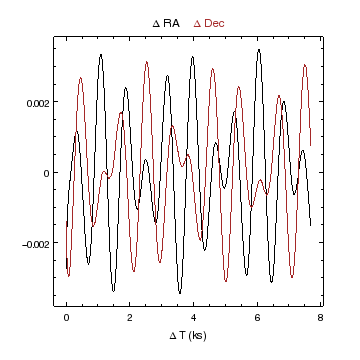The get_col routine can be used to access some of the metadata about a column: for instance
```chips> cd = get_col(cr, "time")
chips> print("Units of time column: %s" % cd.unit)Units of time column: s
```
Unfortunately I have not yet written the documentation to let you really exploit this!Note that unit is a field or attribute of an object (in this case the thing that is returned by get_col), and it is should be considered to be a variable rather than a function - i.e. don't say get_col(cr,"time").unit() otherwise you will see cryptic messages like:
`TypeError: 'str' object is not callable`

If you want to do this for multiple files then you can write a routine to automate this. In this case we want to give a file name and create the plot, so we want something like:

1. `"""Plot up aspect solutions.`
2. `At present, this is a pretty empty module, containing`
3. `only the deltaplot routine.`
4. `"""`
5. `from pycrates import *`
6. `from pychips import *`
7. `from pychips.hlui import *`
8. `import pychips.advanced as adv`
9. `__all__ = ("deltaplot", )`
10. `def deltaplot(filename, color="brown"):`
11. ` """Plot up the changes in ra and dec versus`
12. ` time (measured as an offset since the start of`
13. ` the file, converted to kiloseconds), using`
14. ` the median level of each column as the base value."""`
15. ` # This will throw an error if the columns do not`
16. ` # exist`
17. ` cr = read_file("%s[cols time, ra, dec]" % filename)`
18. ` tunit = get_col(cr, "time").unit`
19. ` if tunit != "s":`
20. ` raise IOError("Units of time column in %s are not seconds but %s" %`
21. ` (filename, tunit))`
22. ` t = copy_colvals(cr, "time")`
23. ` xt = (t - t) / 1e3`
24. ` ra = copy_colvals(cr, "ra")`
25. ` dec = copy_colvals(cr, "dec")`
26. ` yra = ra - np.median(ra)`
27. ` ydec = dec - np.median(dec)`
28. ` # Run all ChIPS commands as if they were a single`
29. ` # command for undo/redo purposes`
30. ` #`
31. ` adv.open_undo_buffer()`
32. ` try:`
33. ` add_curve(xt, yra, ["symbol.style", "none"])`
34. ` add_curve(xt, ydec, ["symbol.style", "none", "line.color", color])`
35. ` set_plot_xlabel(r"\Delta T (ks)")`
36. ` set_plot_title(r"\Delta RA \color{%s}{\Delta Dec}" % color)`
37. ` except:`
38. ` # Throw out all the ChIPS commands and then`
39. ` # re-throw the error`
40. ` adv.discard_undo_buffer()`
41. ` raise`
42. ` # We have finished the ChIPS commands, so close the buffer`
43. ` # so that they are displayed`
44. ` adv.close_undo_buffer()`This line defines what names are exported when the module is imported using the from asolplot import * syntax. For this example there is only one symbol(*) - the deltaplot routine - but often you will have multiple routines, some of which are only necessary in specialized cases. There's also our friend the comma, which is needed here since we again have a tuple with only one element.
(* actually, this isn't quite true, since we also have all the names from the ChIPS and Crates modules that we loaded in)The deltaplot has one required (positional) argument - the name of the file to plot - and one optional (named) argument that declares the color to draw the Dec data. If no second argument is given then the default value of brown is used.Since we convert the time from seconds to an offset in kiloseconds, we check that the TIME column in the input file has the correct units, otherwise we throw an IOError which will exit the routine. In real-life cases you would probably also want to allow columns with no unit information, or just ignore the check and assume you are always going to use the same sort of input file.Here we use a routine from the advanced ChIPS module to make it look to the user that all the ChIPS calls we make are acually a single command. This allows a user to undo the plot creation with a single call to undo, and means that we do not have to bother with adjusting the window.display setting. Since we are in an "undo buffer", we need to make sure that we always either close it (using adv.close_undo_buffer) if everything succeeded, or discard it with adv.discard_undo_buffer if there was an error. In the latter case, we re-throw the error, using the raise statement, so that the user knows what went wrong.

If asolplot.py is in your current directory, then you can load it as if it were a module (that's because it is!):

```chips> import asolplot as ap
chips> help apHelp on module asolplot:

NAME
asolplot - Plot up aspect solutions.

FILE
/Users/dburke/ciao/talks/ciao-workshop-2010/scripting/asolplot.py

DESCRIPTION
At present, this is a pretty empty module, containing
only the deltaplot routine.

FUNCTIONS
deltaplot(filename, color='brown')
Plot up the changes in ra and dec versus
time (measured as an offset since the start of
the file, converted to kiloseconds), using
the median level of each column as the base value.

DATA
__all__ = ('deltaplot',)

chips> ap.deltaplot("asol1.fits")
chips> erase()
chips> ap.deltaplot("asol1.fits", "green")chips> erase()
chips> ap.deltaplot("asol1.fits", color="red")
chips> erase()
chips> ap.deltaplot("asol1.fits", color="green-with-yellow-polkadots")chips ERROR: The color 'green-with-yellow-polkadots' is unknown or not supported
```Here we see why we wrote the documentation strings in the Python code: we can use help to remind ourselves what a particular bit of code does! Here we get the documentation on the module as a whole; help ap.deltaplot would give the help for the deltaplot routine only.The optional argument can be given as if it were a positional argument or using its name.Using an incorrect color is only caught in the second add_curve command, at line 41, but it appears as if it is a normal ChIPS error to the user, and no half-finished plot appears.If the commands are generally useful, you may want to place them in a more accessible location and then add that directory to your PYTHONPATH environment variable. This is infact how the chips_contrib module we used earlier is made available (although in this case the CIAO startup script adds in the necessary directories to the system set up so you don't have to).

### Playing with images

Here we are going to read in and then display an image. The support for images in ChIPS is new in CIAO 4.2 and is missing some useful capabilites, such as using anything but a linear scale for the pixel values. However, we can manually transform the image to the scale we want, and then display that, so let's see how we go about that.

```chips> cr = read_file("a2142_smoothed.fits")
```

which doesn't provide a great view of all the data in this image (we show the image below). We therefore want to try other scalings, such as logarithmic. First we need to get the pixel data out of the Crate:

```chips> ivals = copy_piximgvals(cr)
chips> ivals.shape
(366, 366)
chips> ivals.min()0.0
chips> ivals.max()
60.028152
chips> ivals.median()AttributeError: 'numpy.ndarray' object has no attribute 'median'
chips> np.median(ivals)
0.27045124769210815
chips> i, = np.where(ivals > 0)ValueError: too many values to unpack
chips> i = np.where(ivals > 0)
chips> i

(array([  0,   0,   0, ..., 365, 365, 365]),
array([147, 148, 149, ..., 219, 220, 221]))
chips> np.median(ivals[i])
1.6809247732162476
```Note that even though we have a two-dimensional image, the min method has returned a scalar value. You can look at the Tentative NumPy Tutorial for more information (e.g. if you want to create the median value per column of an image).Argh! I need to use np.median instead, as the following line shows. You may - or may not - find the error message useful here.Argh! How come my advice about including the "," has failed here? Well, this is a two-dimensional array - as shown by the contents of the shape field above - which means we get back two index arrays. Fortunately we can use the return value to index into the array, but we have to be aware of what data we have if we want to inspect the quantities within the i variable.

Now we can compare several different scalings: linear, logarithmic, and asinh:

```chips> clear()
chips> split(2, 2, 0.1, 0.1)
chips> set_plot_title("linear")
chips> current_plot("plot2")
chips> set_plot_title("log10")
chips> current_plot("plot3")
chips> set_plot_title("asinh")
chips> delete_plot("plot4")
```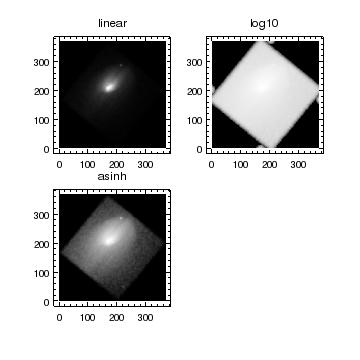The logarithmic scaling shows the image wrap around from smoothing the image using the edges=wrap setting of aconvolve (the "secondary" blobs at the corners of each chip).

So, the asinh scaling gives a nice view of the dynamic range of the data, but we have lost the coordinate information of the axes (the plots above use the pixel coordinates). We need the coordinate information that is stored in the crate (i.e. cr); one way of doing this is to replace the image values with their scaled values in the crate itself:

```chips> cr = read_file("a2142_smoothed.fits")
chips> ivals = copy_piximgvals(cr)
chips> set_piximgvals(cr, np.arcsinhh(ivals))
1
chips> set_xaxis(["tickformat", "ra"])
chips> set_yaxis(["tickformat", "dec"])
chips> set_image(["colormap", "rainbow"])
```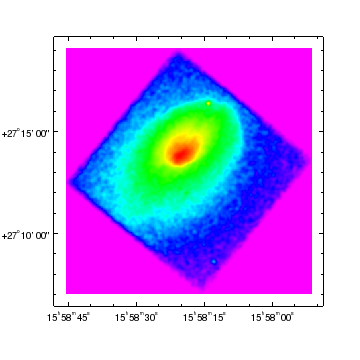This could be automated - made into a routine - and it may well make it into the chips_contrib module at some point in the future. It could look something like:

1. `import pycrates`
2. `import pychips.all as chips`
3. `import numpy as np`
4. `__all__ = ("imgscale", )`
5. `def imgscale(filename, scaling="arcsinh", cmap="rainbow"):`
6. ` """Display the image in filename using the given`
7. ` scaling and colormap (cmap).`
8. ` The value for scaling should be the name of any routine`
9. ` provided by numpy which has the form 'y = np.funcname(x)'.`
10. ` """`
11. ` if hasattr(np, scaling):`
12. ` func = getattr(np, scaling)`
13. ` else:`
14. ` raise AttributeError("There is no numpy routine called '%s'" %`
15. ` scaling)`
16. ` cr = pycrates.read_file(filename)`
17. ` ivals = pycrates.copy_piximgvals(cr)`
18. ` nvals = func(ivals)`
19. ` dummy = pycrates.set_piximgvals(cr, nvals)`
20. ` if dummy != 1:`
21. ` raise StandardError("Unable to change pixel values in crate. Weird!")`
22. ` chips.open_undo_buffer()`
23. ` try:`
24. ` chips.add_image(cr, ["colormap", cmap])`
25. ` chips.set_xaxis(["tickformat", "ra"])`
26. ` chips.set_yaxis(["tickformat", "dec"])`
27. ` except:`
28. ` chips.discard_undo_buffer()`
29. ` raise`
30. ` chips.close_undo_buffer()`This loads in all the ChIPS routines so I don't have to remember whether commands are in the advanced or hlui modules.We could have just used this line, since it throws an error if scaling is invalid, but I wanted to create a slightly-different error message.Here we call the function, using the variable func which we set up earlier. Yay for dynamic programming.